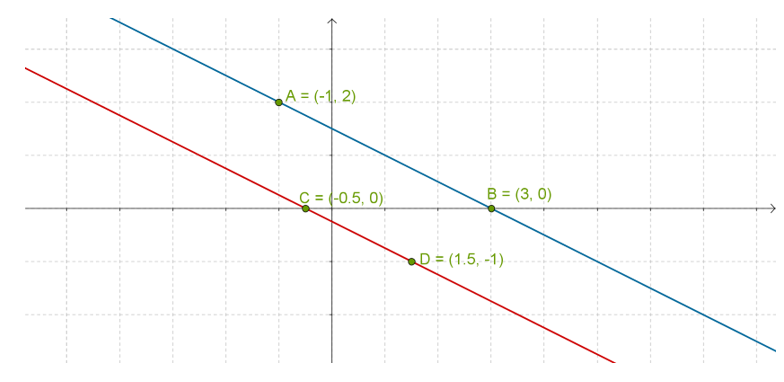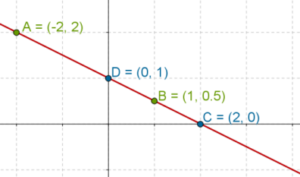Consider the following pair of linear equations:

$\begin{array}{l}x + 2y - 3 = 0\\2x + 4y + 1 = 0\end{array}$

Let us plot the lines corresponding to these equations on the same axes (we have plotted two points on each line):What do we observe? We see that the two lines are parallel, which means that they have no point in common. In other words, there is no pair of values of x and y which can satisfy both linear equations of the pair. In such cases, we say that the pair of linear equations has no solution. Graphically, this means that the corresponding lines are parallel.

Now, consider the following pair of linear equations:

$\begin{array}{l}x + 2y - 2 = 0\\3x + 6y - 6 = 0\end{array}$

Two points satisfying the first equation will be:

$\begin{array}{l}A \equiv \left( { - 2,\;2} \right)\\B \equiv \left( {1,\;0.5} \right)\end{array}$

Two solutions to the second equation will be:

$\begin{array}{l}C \equiv \left( {2,\;0} \right)\\D \equiv \left( {0,\;1} \right)\end{array}$

Now, observe what happens if we plot these points and draw the two lines:They turn out to be the exact same lines! What is happening? One way to look at it is to see that you can take out a common factor of 3 from the second equation, and it becomes identical to the first equation:

$\begin{array}{l}3x + 6y - 6 = 0\\ \Rightarrow \;\;\;3\left( {x + 2y - 2} \right) = 0\\ \Rightarrow \;\;\;x + 2y - 2 = 0\end{array}$

Essentially, the two equations are the same, so obviously, the corresponding lines will also be the same. Technically, we can also say that the pair of linear equations has infinitely many solutions, because all the points on these lines are common to each other.

Pair of Linear Equations in 2 Variables
Pair of Linear Equations in 2 Variables
grade 10 | Questions Set 2
Pair of Linear Equations in 2 Variables
Pair of Linear Equations in 2 Variables
grade 10 | Questions Set 1